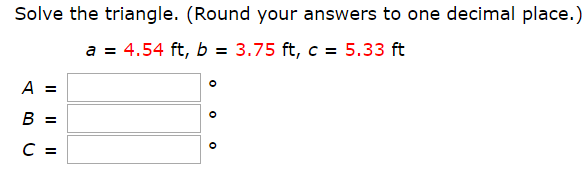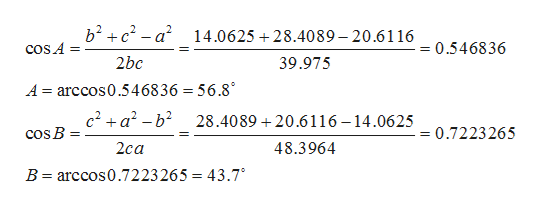# Solve the triangle. (Round your answers to one decimal place.)a = 4.54 ft, b = 3.75 ft, c = 5.33 ftA =B =С

Question
1 views

Photo attachedhelp_outlineImage TranscriptioncloseSolve the triangle. (Round your answers to one decimal place.) a = 4.54 ft, b = 3.75 ft, c = 5.33 ft A = B = С fullscreen
check_circle

Step 1

The length of sides of triangle are given below

Step 2

By, using cosine formul, we find measure of angle A and Bhelp_outlineImage Transcriptioncloseb2ca14.0625 +28.4089 - 20.6116 0.546836 cOS A 2bc 39.975 A arccos0.5468 36 = 56.8 c2+a2-b2 28.408920.6116-14.0625 + cos B = 0.7223265 = 2cа 48.3964 B arccos0.7223 265 43.7 fullscreen
Step 3

Angle C can be evalu...

### Want to see the full answer?

See Solution

#### Want to see this answer and more?

Solutions are written by subject experts who are available 24/7. Questions are typically answered within 1 hour.*

See Solution
*Response times may vary by subject and question.
Tagged in

### Trigonometric Ratios